• ### RMS vs FFT complex magnitudes

If it turns out to be possible to measure how much a signal aliases using an oversampled FFT I think I need to know how to sum the complex magnitudes of an FFT and how that sum relates to the signal's RMS amplitude. I'm trying to figure it out with this patch: compareTimeFreqAmpl.pd .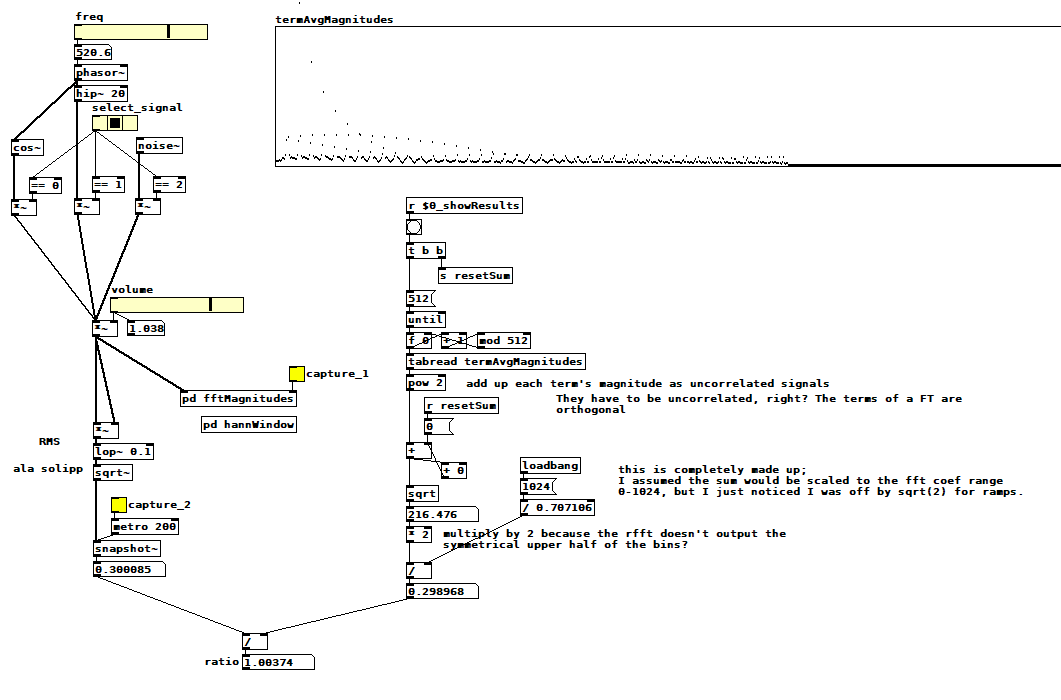Select one of the three signals, pick a frequency if applicable, pick a volume, then capture the average magnitude of each FFT term (it computes the magnitude of the sum of all terms when you clear the capture toggle). Next, capture the RMS amplitude, which displays the ratio between the two measurements as a side effect. I hacked the ratio to be close to 1 for cos~ and ramps, but it's ~1.125 for noise. Note that I'm not using a hann window inside the fft subpatch--I think it's not relevant to this problem, and it seems to only change the ratio by 3/2, which is the expected effect of a hann window with 4x overlap.

You can see my ratio hack in the divide operation just before the ratio computation. I saw that I was off by sqrt(2) so I just included it, but is it sqrt(2), and if so, why? Why is noise different? Is it right to sum the FFT term magnitudes as uncorrelated signals? Is it right to double the sum because the rfft skips the symmetrical upper half of the terms for efficiency? What else am I doing wrong?

Edit: Hmm, that symmetrical upper half of terms? Probably uncorrelated as well. So the doubling has to happen before the sqrt, and then you get...sqrt(2)! Is that plausible?

• Posts 7 | Views 1539
• I tested with a DC signal and was off by another sqrt(2), and that's when I remembered that the DC component isn't doubled. I moved the conditional doubling ahead of the summation which solved my previous off-by-sqrt(2) issue, but I'm still off by an eighth when measuring noise. Why? And why is this stuff so hard?!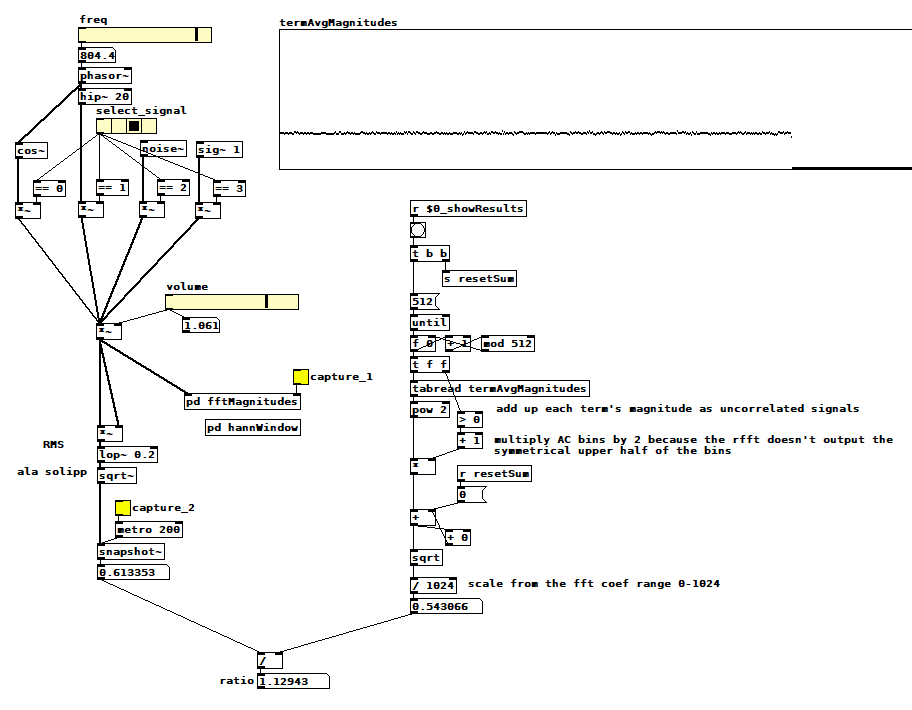compareTimeFreqAmpl.pd

• Today's refinements: it turns out that if N is the window size, [rfft~] returns N/2+1 complex coefficients! Gosh, and there it is, right in the help patch! That means I haven't been including the highest frequency explicit term in the magnitude sum. Together with the fact that white noise has stronger upper partials than cosines and ramps, I'm certain that that omission explains why my noise measurement is low!!!

Nope. Makes no difference. To pile on even further, I later discovered that that last term's magnitude, like the DC term, should not be doubled (more on this after the picture). Here are the latest changes to the patch: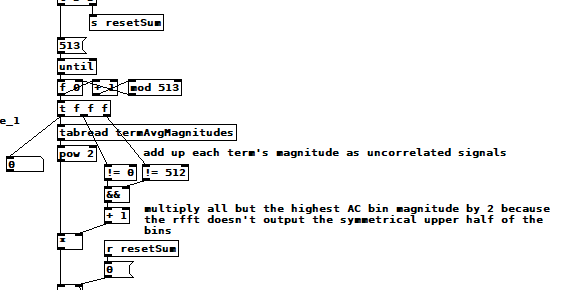compareTimeFreqAmpl.pd

OK, here's another toy I built which helped me discover the N/2+1 term and how to use it. It performs an inverse real FFT by tediously accumulating all the sinusoids at the frequencies, amplitudes, and phase angles determined by the FFT, additive synthesis style. You can wave your hands and theorize all you want but the rubber hits the road when you gotta code it. The result is super fun (to me) because you can "animate" the sum and watch it converge on the original input signal. It feels kind of miraculous. No matter how ef'd up the input signal is, [rfft~] can tell you how to reproduce it using DC and a bunch of sines! 19th c mathematics!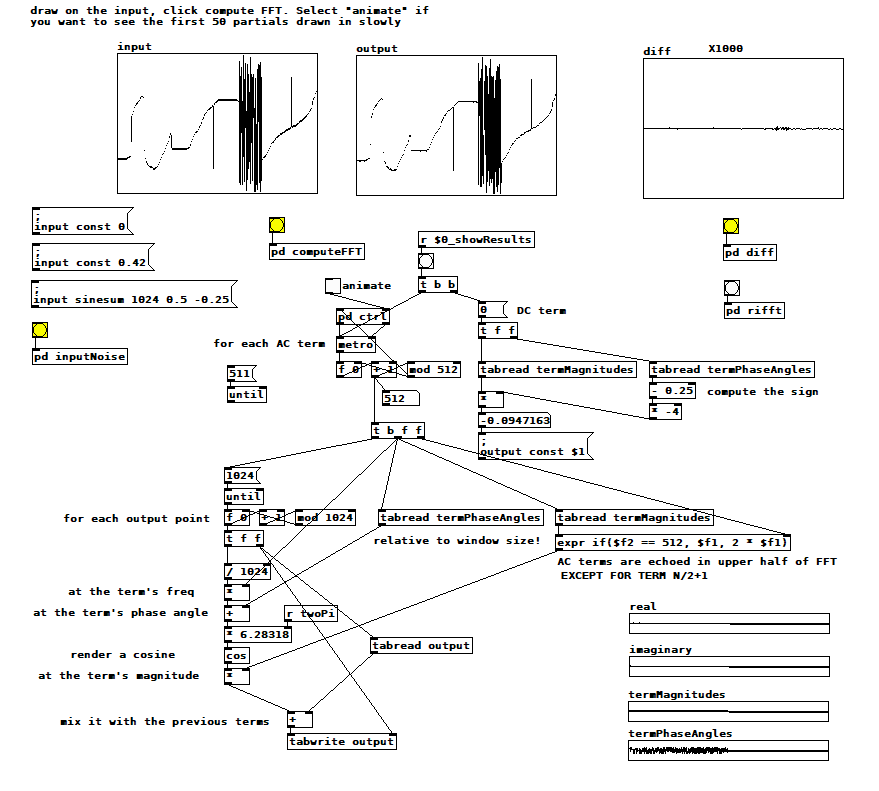manual reverse FT.pd

• Here's another version of the inverse FT using the complex coefficients in rectangular form rather than polar form. It doesn't shed additional light on my original problem, but it's interesting to see the phase angles rendered implicitly. For some reason, you have to reverse the output. Also note that I'm adding sine to cosine, while most texts I've found show sine subtracted from cosine. Why and why?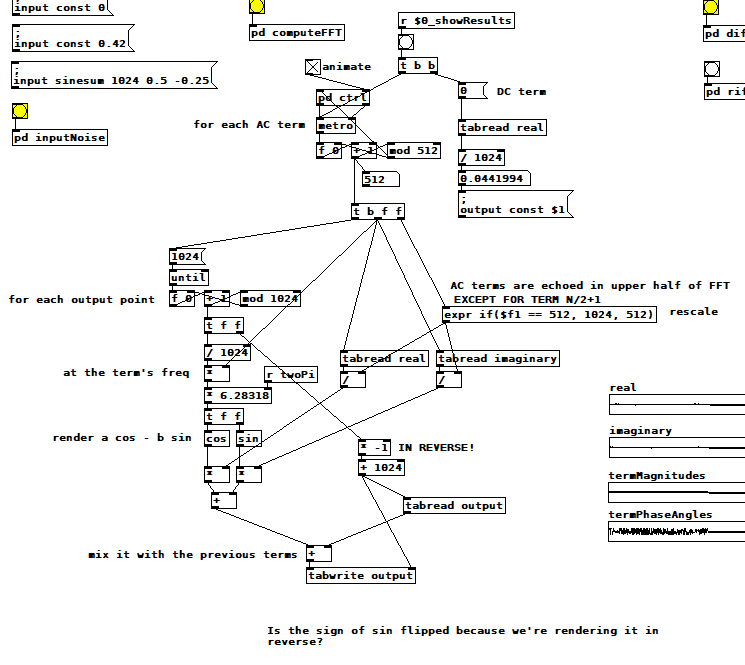manual inverse FT 2.pd

For those following along at home, here's a summary of what I still don't know:
-Why the RMS noise measurment is ~1.13 times the sum of the FFT term magnitudes
-Why some FFT term magnitudes are doubled and others not
-Why, in the polar version of the inverse FT, phase angles are all relative to the whole window (i.e. term 1's sinusoid) rather than to each term's sinusoid
-Why, in the rectangular version, the output is reversed, and why the sine is added instead of subtracted
-If any of the "conclusions" I've drawn from these tests are in fact wrong (e.g. is windowing really not relevant? After all, "no window" is a rectangular window, isn't it?)

• @jameslo @katjav did a long analysis of whys and wherefores of FFT (that I have been intending to read thoroughly for a long time) here....... http://www.katjaas.nl/FFToutput/FFToutput.html
That's a link to the middle of it.
What it does and why it might not do what you expect.
David.

• @whale-av Wow, you're the second person to recommend that blog to me. I'm going to stop posting my muddled thinking and read the whole thing from the beginning. Thanks!

• I'm up to the beginning of Katja V's Fourier Transform section and I've already found a few answers to my questions. I also managed to get the sum of FFT term amplitudes to match the RMS value for arbitrary input. Here's the patch: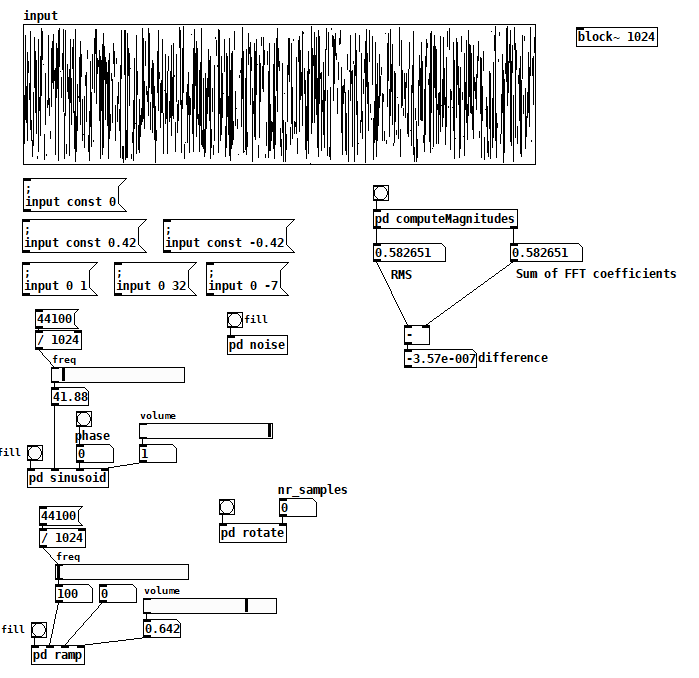Inside [pd computerMagnitudes]: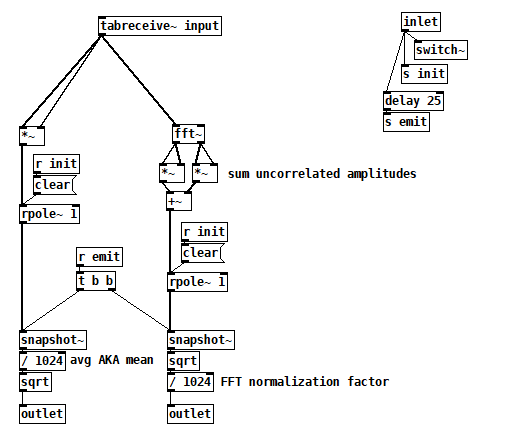compareTimeFreqAmpl2.pd
All the things on the left are just tools to fill the input table, but you can also just draw. Once you have your signal, bang computeMagnitudes to measure its amplitude both ways.

I made a couple of simplifications that not only got the test working but also gave me more confidence that I was comparing apples to apples:

• I'm computing RMS and the FFT from a single static 1024 vector, so I'm now comparing two views of the exact same signal and there's no need for averaging.
• I learned from Katja that if you perform a complex FFT on a real signal, you don't have to worry about which terms to double because the FFT gives you those terms's double in the upper half of the output explicitly. The real FFT skips the upper half for efficiency because it's related to the lower half.
• I also learned that even the cosine and sine components of each harmonic are uncorrelated signals, so I now sum their magnitudes individually across all harmonics. There's no need to compute the magnitude of each FFT term first.

So I think the issue I was having with noise was just an artifact of a badly programmed test, probably having to do with the way I was averaging term magnitudes, but I don't really know.

7/18/2020 update: I've found info in Katja's blog that suggests that this patch is wrong (or maybe even not possible). Exhibit A: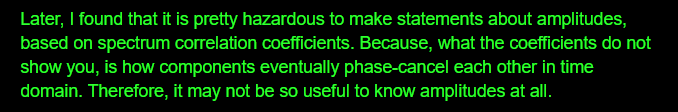IMHO, this contradicts what she spent so much effort establishing on the prior two pages (http://www.katjaas.nl/sinusoids2/sinusoids2.html
http://www.katjaas.nl/correlation/correlation.html), that the cos and sine components of all FFT terms are orthogonal. If they're orthogonal, how could they cancel each other out?

She raises a another point on the FFT output page that really makes me wonder why my patch seems to work: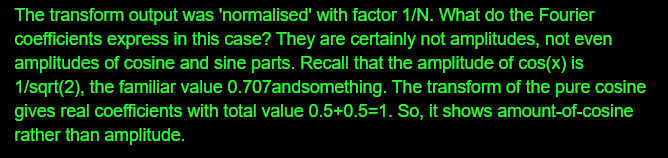In this case I agree--Fourier coefficents are really the peak amplitudes of the cos and sine components--but my confusion over this is what made me program the patch the way that I did. So why is it working?

Posts 7 | Views 1539
Internal error.

Oops! Looks like something went wrong!